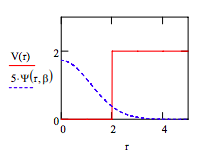Skip to main content

# 432: Variation Method for a Particle in a Finite 3D Spherical Potential Well

This problem deals with a particle of unit mass in a finite spherical potential well of radius 2 ao and well height 2 Eh. The trial wave function is given below.

$\psi (r, \beta ) := ( \frac{2 \beta}{ \pi})^{ \frac{3}{4}} exp(- \beta r^2)$

$T = - \frac{1}{2r} \frac{d^2}{dr^2} (r \blacksquare )$

$$V(r) := if [(r \leq 2), 0, 2]$$a. Demonstrate that the wave function is normalized.

$\int_{0}^{ \infty} \psi (r, \beta )^2 4 \pi r^2 dr |_{simplify}^{assume,~ \beta > 0} \rightarrow 1$

b. Evaluate the variational integral.

$E( \beta ) := \int_{0}^{ \infty} \psi (r, \beta ) [- \frac{1}{2r} \frac{d^2}{dr^2} (r \psi (r, \beta ))] 4 \pi r^2 dr ... |_{simplify}^{assume,~ \beta > 0} + \int_{2}^{ \infty} 2 \psi (r, \beta )^2 4 \pi r^2 dr$

$E( \beta ) := \frac{1}{2} \frac{3 \pi^{ \frac{1}{2}} \beta + 4 \pi ^{ \frac{1}{2}} + 16 exp(-8 \beta) 2^{ \frac{1}{2}} \beta^{ \frac{1}{2}}-4 \pi ^{\frac{1}{2}} erf((2) 2^{ \frac{1}{2}} \beta^{ \frac{1}{2}}) }{ \pi ^{ \frac{1}{2}}}$

c. Minimize the energy with respect to the variational parameter $$\beta$$.

$$\beta$$ := 5 $$\beta$$ := Minimize (E, \beta ) $$\beta$$ = 0.381 $$E ( \beta ) = 0.786$$

d. Calculate the average value of r.

$$\int_{0}^{ \infty} r \psi (r, \beta )^2 4 \pi r^2 dr = 1.293$$

e. Calculate the kinetic and potential energy.

Potential energy:

$$\int_{2}^{ \infty} r \psi (r, \beta )^2 4 \pi r^2 dr = 0.215$$

Kinetic energy:

$$E( \beta ) - 0.215 = 0.571$$

f. Calculate the probability that the particle is in the barrier.

$$1 - \int_{0}^{2} \psi (r, \beta )^2 4 \pi r^2 dr = 0.107$$

g. Plot the wavefunction on the same graph as the potential energy.• Was this article helpful?